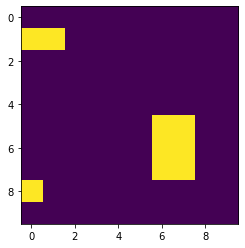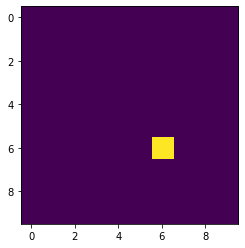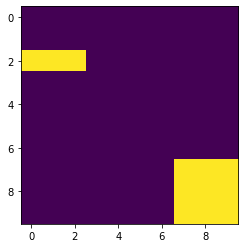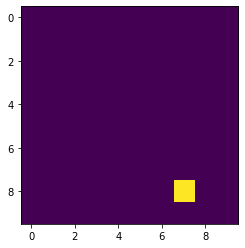# Mahotas – Hit & Miss transform

• Last Updated : 10 Jul, 2020

In this article we will see how we can perform hit and miss transform in mahotas. In mathematical morphology, hit-or-miss transform is an operation that detects a given configuration in a binary image, using the morphological erosion operator and a pair of disjoint structuring elements.

In order to do this we will use `mahotas.hitmiss` method

Syntax : mahotas.hitmiss(img, template)

Argument : It takes two numpy ndarray as argument

Return : It returns ndarray

Below is the implementation

 `# importing required libraries``import` `mahotas as mh``import` `numpy as np``from` `pylab ``import` `imshow, show``   ` `# creating region``# numpy.ndarray``regions ``=` `np.zeros((``10``, ``10``), ``bool``)``   ` `# setting 1 value to the region``regions[``1``, :``2``] ``=` `1``regions[``5``:``8``, ``6``: ``8``] ``=` `1``regions[``8``, ``0``] ``=` `1``     ` `# showing the image with interpolation = 'nearest'``print``(``"Image"``)``imshow(regions, interpolation ``=``'nearest'``)``show()``  ` `# template for hit miss``template ``=` `np.array([``            ``[``0``, ``1``, ``1``],``            ``[``0``, ``1``, ``1``],``            ``[``0``, ``1``, ``1``]])``  ` `# hit miss transform``img ``=` `mahotas.hitmiss(regions, template)`` ` `# showing image``print``(``"Image after hit miss transform"``)``imshow(img)``show()`

Output :

`Image``Image after hit miss transform`Another example

 `# importing required libraries``import` `mahotas as mh``import` `numpy as np``from` `pylab ``import` `imshow, show``   ` `# creating region``# numpy.ndarray``regions ``=` `np.zeros((``10``, ``10``), ``bool``)``   ` `# setting 1 value to the region``regions[``2``:``3``, :``3``] ``=` `1``regions[``7``:, ``7``:] ``=` `1``     ` `# showing the image with interpolation = 'nearest'``print``(``"Image"``)``imshow(regions, interpolation ``=``'nearest'``)``show()``  ` `# template for hit miss``template ``=` `np.array([``            ``[``0``, ``1``, ``1``],``            ``[``0``, ``1``, ``1``],``            ``[``0``, ``1``, ``1``]])``  ` `# hit miss transform``img ``=` `mahotas.hitmiss(regions, template)`` ` `# showing image``print``(``"Image after hit miss transform"``)``imshow(img)``show()`

Output :

`Image``Image after hit miss transform`My Personal Notes arrow_drop_up